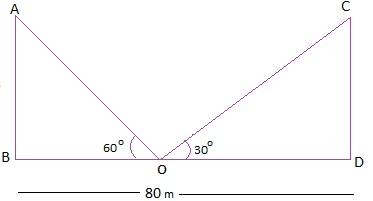Guru

# Two poles of equal heights are standing opposite each other on either side of the road, which is 80 m wide. From a point between them on the road, the angles of elevation of the top of the poles are 60° and 30°, respectively. Find the height of the poles and the distances of the point from the poles. Q.10

• 0

How i solve this problem of Applications of Trigonometry of class 10th ncert math of exercise 9.1 of question no.10, sir give me the best way to solve this problem Two poles of equal heights are standing opposite each other on either side of the road, which is 80 m wide. From a point between them on the road, the angles of elevation of the top of the poles are 60° and 30°, respectively. Find the height of the poles and the distances of the point from the poles.

Share

1. Let AB and CD be the poles of equal height.

O is the point between them from where the height of elevation taken. BD is the distance between the poles.As per above figure, AB = CD,

OB + OD = 80 m

Now,

In right ΔCDO,

tan 30° = CD/OD

1/√3 = CD/OD

CD = OD/√3 … (1)

Again,

In right ΔABO,

tan 60° = AB/OB

√3 = AB/(80-OD)

AB = √3(80-OD)

AB = CD (Given)

√3(80-OD) = OD/√3 (Using equation (1))

3(80-OD) = OD

240 – 3 OD = OD

4 OD = 240

OD = 60

Putting the value of OD in equation (1)

CD = OD/√3

CD = 60/√3

CD = 20√3 m

Also,

OB + OD = 80 m

⇒ OB = (80-60) m = 20 m

Thus, the height of the poles are 20√3 m and distance from the point of elevation are 20 m and

60 m respectively.

• 0